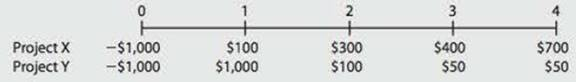Chapter 11, Problem 13PFundamentals of Financial Manageme...

14th Edition
Eugene F. Brigham + 1 other
ISBN: 9781285867977

Solutions

Chapter
SectionFundamentals of Financial Manageme...

14th Edition
Eugene F. Brigham + 1 other
ISBN: 9781285867977
Textbook Problem

MIRR A firm is considering two mutually exclusive projects, X and Y, with the following cash flows:The projects are equally risky, and their WACC is 12%. What is the MIRR of the project that maximizes shareholder value?

Summary Introduction

To determine: The MIRR of the project which increases the shareholders’ value.

Modified internal rate of return (MIRR):

Modified internal rate of return holds an assumption that the positive cash flows should be invested again in the firm's cost of capital, and the initial expenses are financed at the firm's financing cost.

Explanation

Step 1: Calculate the total terminal values of the cash flows of Project X.

 Year Cash flows Future value at 12% Terminal values 0 -1,000 0 0 1 100 1.4049 140.49 2 300 1.2544 376.32 3 400 1.12 448 4 700 1 700 Total terminal values 1,664.81

Therefore, the total terminal value is $1,664.81. Step 2: Calculate the modified internal rate of return of Project X. MIRR=TerminalvalueOutlayn1=$1,664.81\$1,00041=1.6648141=0.1359or13.59%

Therefore, the modified internal rate of return is 13.59%.

Step 3: Calculate the total terminal values of the cash flows of Project Y

Still sussing out bartleby?

Check out a sample textbook solution.

See a sample solution

The Solution to Your Study Problems

Bartleby provides explanations to thousands of textbook problems written by our experts, many with advanced degrees!

Get Started